Warning: Illegal string offset 'html' in /home/hsn/public_html/forum/cache/skin_cache/cacheid_1/skin_topic.php on line 909

1 + 1 = 1 ? ? ? - HSN forum# 1 + 1 = 1 ? ? ?

11 replies to this topic

### #1cK_hoangan

• Gender:Not Telling

Posted 28 November 2007 - 10:29 PM

This is a "trick stuff" in Maths which quite interesting. Hope that anyone will enjoy it.

Assume : A = B
=> A^2 = AB (multiply both by A)
=> A^2 - B^2 = AB - B^2 (take away both to B^2)
=> (A-B)(A+B)= B (A-B)
=> A+B = B
=> 2B = B (hence A = B)
=> 2 = 1
=> 1 + 1 = 1BINGO !!!

I think we shouldn't study Maths anymore cause It's all wrong now ...### #2Jammie1000000

• Gender:Male

Posted 29 November 2007 - 01:31 PM

This is a "trick stuff" in Maths which quite interesting. Hope that anyone will enjoy it.

Assume : A = B
=> A^2 = AB (multiply both by A)
=> A^2 - B^2 = AB - B^2 (take away both to B^2)
=> (A-B)(A+B)= B (A-B)
=> A+B = B
=> 2B = B (hence A = B)
=> 2 = 1
=> 1 + 1 = 1BINGO !!!

I think we shouldn't study Maths anymore cause It's all wrong now ...(A-B)=0 so you cannot divide through by (A-B) as you cannot divide by 0### #3cK_hoangan

• Gender:Not Telling

Posted 29 November 2007 - 01:45 PM

Well-done !!!!as I said, it's all for relaxing :D

You wanna try once more with 1 > 4 ? I will prove it soon### #4Marcus

Site Swot

•• Members
•• 147 posts
• Location:Aberdeen
• Interests:Guitar - Passed my grade 6 :D :D<br />Write computer programs - won Heriot-Watt programming challenge 2006 and 2007 :D<br />
• Gender:Male

Posted 06 January 2008 - 04:01 PM

just to go along the same lines of posting bizarre mathematics, here is a proof that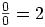or in fact any number you wanteqn 1) x+1 = 0
eqn 2) 2x+2 = 0

divide eqn 2 by eqn 1cancel the x+1 on top and bottom to get: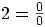but this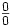= anything helps prove the above problem...

=> (A-B)(A+B)= B (A-B) , (A-B) = 0
=> 0(A+B)= 0B
=>2B =B
so if=2
then this works XD XD
=-=-=Marcus=-=-=

Top of the Class

•• Members
•• 390 posts
• Location:Cambridge
• Interests:Muzak.
• Gender:Male

Posted 07 January 2008 - 07:44 PM

Here's a tough one (you'll need to have done complex numbers, can't remember when it comes up in advanced higher):Where's the error?

HMFC - Founded 1874, beefing the Cabbage since 1875

### #6Marcus

Site Swot

•• Members
•• 147 posts
• Location:Aberdeen
• Interests:Guitar - Passed my grade 6 :D :D<br />Write computer programs - won Heriot-Watt programming challenge 2006 and 2007 :D<br />
• Gender:Male

Posted 10 January 2008 - 11:43 PM

Right I don't think this is correct but: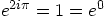and also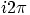is usually written asas angles usually lie between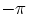and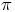so when you multiplied theby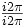you were effectively multiplying bywhich you can't do.

Am i even remotely on the right lines here?=-=-=Marcus=-=-=

### #7englisher

• Location:5th Year
• Gender:Male

Posted 21 February 2008 - 02:55 PM

Here's a tough one (you'll need to have done complex numbers, can't remember when it comes up in advanced higher):Where's the error?

Can't put brackets round e^2ipi ?

### #8Marcus

Site Swot

•• Members
•• 147 posts
• Location:Aberdeen
• Interests:Guitar - Passed my grade 6 :D :D<br />Write computer programs - won Heriot-Watt programming challenge 2006 and 2007 :D<br />
• Gender:Male

Posted 22 February 2008 - 09:16 PM

Can't put brackets round e^2ipi ?

I wish it was as simple as that, but yeah you can... one of the rules of indices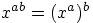in the case of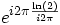a would beand b would be=-=-=Marcus=-=-=

### #9Wildespleen

• Gender:Male

Posted 21 January 2009 - 08:13 PM

Can I just confirm that e^2ipi is indeed one? I presume it originates from 'e^ipi + 1 = 0'? (Which we haven't really covered in class so far, we only mentioned it).
I've puzzled over this one for a while now and the only real possible fault I, personally, can find is this. Failing that, I fear the textbooks will have to be rewritten-> 1+1=1 and 2+2=2! There'll be anarchy in the streets! XD

### #10Marcus

Site Swot

•• Members
•• 147 posts
• Location:Aberdeen
• Interests:Guitar - Passed my grade 6 :D :D<br />Write computer programs - won Heriot-Watt programming challenge 2006 and 2007 :D<br />
• Gender:Male

Posted 27 January 2009 - 02:12 PM

QUOTE (Wildespleen @ Jan 21 2009, 08:13 PM) <{POST_SNAPBACK}>
Can I just confirm that e^2ipi is indeed one? I presume it originates from 'e^ipi + 1 = 0'? (Which we haven't really covered in class so far, we only mentioned it).
I've puzzled over this one for a while now and the only real possible fault I, personally, can find is this. Failing that, I fear the textbooks will have to be rewritten-> 1+1=1 and 2+2=2! There'll be anarchy in the streets! XD

Yeah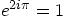, and it comes from Euler's formula: (only briefly mentioned in AH)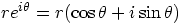I had to ask my uni profs to explain what is wrong with this proof...
=-=-=Marcus=-=-=

### #11Monkey Man

• Location:Glasgow
• Gender:Male

Posted 02 January 2010 - 06:50 PM

QUOTE Jammie1000000
QUOTE(cK_hoangan @ Nov 28 2007, 10:29 PM) View Post
This is a "trick stuff" in Maths which quite interesting. Hope that anyone will enjoy it.

Assume : A = B
=> A^2 = AB (multiply both by A)
=> A^2 - B^2 = AB - B^2 (take away both to B^2)
=> (A-B)(A+B)= B (A-B)
=> A+B = B
=> 2B = B (hence A = B)
=> 2 = 1
=> 1 + 1 = 1 blink.gif BINGO !!!

I think we shouldn't study Maths anymore cause It's all wrong now ... tongue.gif

(A-B)=0 so you cannot divide through by (A-B) as you cannot divide by 0

cool.gif

can i just add that this is not dividing through by (A-B), they cancel each other out### #12Marcus

Site Swot

•• Members
•• 147 posts
• Location:Aberdeen
• Interests:Guitar - Passed my grade 6 :D :D<br />Write computer programs - won Heriot-Watt programming challenge 2006 and 2007 :D<br />
• Gender:Male

Posted 27 January 2010 - 05:37 PM

but they cancel out, because you are dividing through by (a-b) << that the operation you are actually doing when you cancel out terms
=-=-=Marcus=-=-=

#### 1 user(s) are reading this topic

0 members, 1 guests, 0 anonymous users